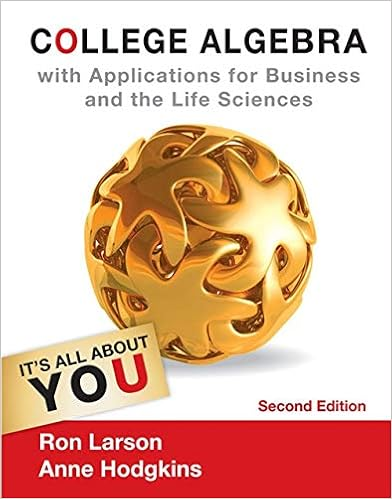# A cost function is a ________

• Test Prep
• 63
• 95% (282) 269 out of 282 people found this document helpful

This preview shows page 1 - 3 out of 63 pages.

##### We have textbook solutions for you!
The document you are viewing contains questions related to this textbook.The document you are viewing contains questions related to this textbook.
Chapter 1 / Exercise 54
College Algebra with Applications for Business and Life Sciences
LarsonExpert Verified
Cost Accounting: A Managerial Emphasis, 16e (Horngren) Chapter 10 Determining How Costs Behave 10.1 Objective 10.1 1) A cost function is a ________. A) process of calculating present value of projected cash flows B) process of allocating costs to cost centers or cost objects C) mathematical description of how a cost changes with changes in the level of an activity relating to that cost D) is a very thorough and detailed way to identifying a cost object when there is a physical relationship between inputs and outputs Answer: C
Diff: 2 Objective: 1 AACSB: Analytical thinking 2) Bennet Company employs 20 individuals. Eighteen employees are paid \$18 per hour and the rest are salaried employees paid \$3,000 a month. Which of the following is the total cost function of personnel? A Diff: 2
Objective: 1 AACSB: Analytical thinking 3) Crimson Services, Inc., employs 8 individuals. They are all paid \$16.50 per hour. How would total costs of personnel be classified? A
Diff: 2 Objective: 1 AACSB: Analytical thinking
##### We have textbook solutions for you!
The document you are viewing contains questions related to this textbook.The document you are viewing contains questions related to this textbook.
Chapter 1 / Exercise 54
College Algebra with Applications for Business and Life Sciences
LarsonExpert Verified
4) For February, the cost components of a picture frame include \$0.40 for the glass, \$0.67 for the wooden frame, and \$0.83 for assembly. The assembly desk and tools cost \$570. Two hundred fifty frames are expected to be produced in the coming year. What cost function best represents these costs? B Diff: 2
Objective: 1 AACSB: Analytical thinking 5) The cost components of a heater include \$35 for the compressor, \$11 for the sheet molded compound frame, and \$74 per unit for assembly. The factory machines and tools cost is \$55,000. The company expects to produce 1,300 heaters in the coming year. What cost function best represents these costs? A) y = 55,000 + 120X B) y = 1,300 + 55,000X C) y = 55,000 + 1,300X D) y = 1,300 + 120X Answer: Explanation: Per unit costs: 35 + 11 + 74 = 120 Diff: 2 A Objective: 1
AACSB: Analytical thinking 6) Which of the following statements is true of a linear cost function? A Diff: 2
Objective: 1 AACSB: Analytical thinking 7) A finance manager has to select one of the four different suppliers of raw materials. The total cost functions under the four options are given below. Assume the quality of the raw material to be the same, which of the following is preferred by the finance manager? C
Diff: 3 Objective: 1 AACSB: Analytical thinking
•••